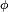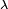# Geographic coordinate system facts for kids

Kids Encyclopedia Facts

A geographical coordinate system is a coordinate system. This means that every place can be specified by a set of three numbers, called coordinates.

A full circle can be divided into 360 degrees (or 360°); this was first done by the Babylonians; Ancient Greeks, like Ptolemy later extended the theory.

Today, degrees are divided further. There are minutes, and seconds; 1 minute (or 1') in this context is 1/60 of a degree; 1 second (or 1") is 1/60 of a minute.

The first concept needed is called latitude (Lat, or the Greek symbol "phi",$\scriptstyle{\phi}\,\!$). For it, the Earth is cut up into 180 circles, from the Equator. The poles are at 90°, the North Pole is at 90° N(orth), the South Pole is at 90° S(outh). Places with the same latitude are on a circle, around the Earth.

The other concept is called longitude (Long, or the Greek symbol "lambda",$\scriptstyle{\lambda}\,\!$), sometimes referred to as "meridian". The 0° longitude line (or zero meridian) goes through the Royal Observatory in Greenwich. Greenwich is a part of London. Then lines are drawn in a similar way; the opposite (or "antipodal") meridian of Greenwich is considered both 180°W(est), and 180°E(ast).

The third number is the height, altitude, or depth. This is given with respect to some fixed (usually easily calculable point). One of these is called mean sea level.

## Images for kidsGeographic coordinate system Facts for Kids. Kiddle Encyclopedia.